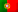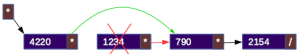Home > Technology > Computer Science > Programming > C++ > Singly linked lists in C++ (Part II)

# Singly linked lists in C++ (Part II)

In the first part of this article, we saw the definition of singly linked lists and methods for inserting nodes. Now, let’s see methods for removing.In the picture above, we can see that, if we want to remove the first node from a list (removing at the head), the process is very easy. We need to access to the first node, which is being pointed by the head, in order to get the pointer for the second node (“next” pointer) and copy it to the head. This way, the head will now be pointing to the second node, which will become the first node. In the end, we free the pointer for the first node from memory. We will see this code together with the removal at the middle of the list.

### Removing at the middle of the list and at the tailIn order to remove a node at the middle of the list, we need to find the node that precedes the node we want to remove. This because we need to have access to the previous node so we can copy the next node pointer from the node we want to remove to that previous one. This way, we can change the next node pointer of the previous node to point to the node that is after the one we want to remove (green arrow). After doing so, we can then free from memory the pointer to the node we want to remove.

Removing a node at the tail can be made exactly by the same way we remove a node at the middle. In this case, the next node pointer of the node we want to remove is set to NULL. So, by copying it to the previous node, we are making it the new last node!

Let’s now take a look on the following code in C++ that will search a node with a given value to remove it.

```    int v = value_to_remove;
bool removed = false;
if (node->value==v) {
free(node);
removed = true;
}
else {
while (node->next!=NULL) {
Node *remove = node->next;
if (remove->value==v) {
node->next = remove->next;
free(remove);
removed = true;
break;
}
node = node->next;
}
}
if (!removed) {
}
```

So, on line 1, we declare an integer variable “v” with the value we want to remove. On line 4, we test if the value is in the node pointed by the head (first node). If it is, we have the case of removing a node at the head! So, on line 5, we change the head to point to the next node (second node) and then we free from memory the node we want to remove. Otherwise, we’ll have to search the node that precedes the node we want to remove in a “while” cycle (line 10). On each iteration, we get the reference to the next node (line 11) to check if it is the one we want to remove (12). When we find it, we copy its next node pointer to the previous node (line 13) and we then free it from memory (line 14). The code will also check if the the value we want to remove was found and displays a message if it wasn’t.

Notice that in this code we did not check if the list is empty. But we should make that test as you will see in the complete code down below.

Meantime, another important note is that we should always free from memory all the nodes that might yet be allocated when we exit the program. One way to do it is to implement a recursive method that will iterate all nodes and then it will start to free each node from the tail to the head:

```void free_list(Node *n) {
if (n!=NULL) {
free_list(n->next);
cout << "Releasing node with the value: " << n->value << endl;
free(n);
}
else {
return;
}
}
```

Before we exit the program, we call this method by sending the head as the parameter!

```    free_list(head);
```

Now, let’s see the complete code:

```#include <iostream>
#include <stdlib.h>

using namespace std;

struct Node {
int value;
Node *next;
};

void free_list(Node *n) {
if (n!=NULL) {
free_list(n->next);
cout << "Releasing node with the value: " << n->value << endl;
free(n);
}
else {
return;
}
}

int main() {

Node *node = NULL;

cout << "1 - Insert a value at the head" << endl;
cout << "2 - Insert a value at the tail" << endl;
cout << "3 - List node values" << endl;
cout << "4 - Remove a node with a value x" << endl;
cout << "0 - Exit program" << endl;

case '0':
break;
case '1':
cout << "Insert a new value at the head of the list: ";
node = (Node*) malloc(sizeof(Node));
node->next = NULL;
}
else {
}
break;
case '2':
char value_for_tail;
cout << "Insert a new value at the tail of the list: ";
cin >> value_for_tail;
node = (Node*) malloc(sizeof(Node));
node->value=atoi(value_for_tail);
node->next = NULL;
}
else {
while (search_last->next!=NULL) {
search_last = search_last->next;
}
search_last->next = node;
}
break;
case '3':
cout << "The list is empty!" << endl;
}
else {
while (node!=NULL) {
cout << "Value: " << node->value << endl;
node = node->next;
}
}
break;
case '4':
cout << "The list is empty!" << endl;
}
else {
char value_to_remove;
cout << "Insert the value to remove: ";
cin >> value_to_remove;
int v = atoi(value_to_remove);
bool removed = false;
if (node->value==v) {
free(node);
removed = true;
}
else {
while (node->next!=NULL) {
Node *remove = node->next;
if (remove->value==v) {
node->next = remove->next;
free(remove);
removed = true;
break;
}
node = node->next;
}
}
if (!removed) {
}
}
break;
default:
cout << "Invalid option!" << endl;
break;
}

}

return 0;

}
```

In the third and final part of this article we will see an example of implementing a list with classes.